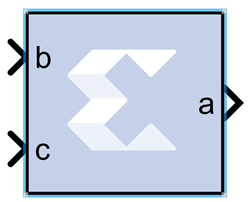# BitBasher - 2020.2 English

## Vivado Design Suite Reference Guide: Model-Based DSP Design Using System Generator (UG958)

Document ID
UG958
Release Date
2020-11-18
Version
2020.2 English

This block is listed in the following Xilinx® Blockset libraries: Basic Elements, Data Types, and Index.

The Xilinx BitBasher block performs slicing, concatenation and augmentation of inputs attached to the block.The operation to be performed is described using Verilog syntax which is detailed in this document. The block can have up to four output ports. The number of output ports is equal to the number of expressions. The block does not cost anything in hardware.

## Block Parameters

The block parameters dialog box can be invoked by double-clicking the icon in your Simulink® model.

Basic tab
Parameters specific to the Basic tab are as follows.
BitBasher Expression
Bitwise manipulation expression based on Verilog Syntax. Multiple expressions (limited to a maximum of 4) can be specified using new line as a separator between expressions.
Output Type tab
Output
This refers to the port on which the data type is specified.
Output type
Arithmetic type to be forced onto the corresponding output.
Binary Point
Binary point location to be forced onto the corresponding output.

Other parameters used by this block are explained in the topic Common Options in Block Parameter Dialog Boxes.

## Supported Verilog Constructs

The BitBasher block only supports a subset of Verilog expression constructs that perform bitwise manipulations including slice, concatenation, and repeat operators. All specified expressions must adhere to the following template expression:

``````
output_var = {bitbasher_expr}
``````

bitbasher_expr: A slice, concat or repeat expression based on Verilog syntax or simply an input port identifier.

output_var: The output port identifier. An output port with the name output_var will appear on the block and will hold the result of the wire expression bitbasher_expr.

Concat

``````
output_var = {bitbasher_expr1, bitbasher_expr2, bitbasher_expr3}
``````

The concat syntax is supported as shown above. Each of bitbasher_exprN could either be an expression or simply an input port identifier.

The following are some examples of this construct:

``````
a1 = {b,c,d,e,f,g}
a2 = {e}
a3 = {b,{f,c,d},e}
``````

Slice

``````
output_var = {port_identifier[bound1:bound2]}(1)
output_var = {port_identifier[bitN]}(2)
``````

port_identifier: The input port from which the bits are extracted.

bound1, bound2: Non-negative integers that lie between 0 and (bit-width of port_identifier – 1)

bitN: Non-negative integers that lie between 0 and (bit-width of port_identifier – 1)

As shown above, there are two schemes to extract bits from the input ports. If a range of consecutive bits need to be extracted, then the expression of the following form should be used.

output_var = {port_identifier[bound1:bound2]}(1)

If only one bit is to be extracted, then the alternative form should be used.

output_var = {port_identifier[bitN]}(2)

The following are some examples of this construct:

``````
a1 = {b[7:3]}
``````

a1 holds bits 7 through 3 of input b in the same order in which they appear in bit b (for example, if b is 110110110 then a1 is 10110).

``````
a2 = {b[3:7]}
``````

a2 holds bits 7 through 3 of input b in the reverse order in which they appear in bit b (for example, if b is 110100110 then a2 is 00101).

``````
a3 = {b}
``````

a3 holds bit 5 of input b.

``````
a4 = {b[7:5],c[3:9],{d,e}}
``````

The above expression makes use of a combination of slice and concat constructs.Bits 7 through 5 of input b, bits 3 through 9 of input `c` and all the bits of `d` and `e` are concatenated.

Repeat

``````
output_var = {N{bitbasher_expr}}
``````

N: A positive integer that represents the repeat factor in the expression

The following are some examples of this construct:

``````
a1 = {4{b[7:3]}}
``````

The above expression is equivalent to a1 = {b[7:3], b[7:3], b[7:3], b[7:3]}

``````
a2 = {b[7:3],2{c,d}}
``````

The above expression is equivalent to a2 = {b[7:3],c,d,c,d}

Constants

Binary Constant: N'bbin_const

Octal Constant: N'ooctal_const

Decimal Constant: N'doctal_const

N: A positive integer that represents the number of bits that are used to represent the constant

bin_const: A legal binary number string made up of 0 and 1

octal_const: A legal octal number string made up of 0, 1, 2, 3, 4, 5, 6 and 7

decimal_const: A legal decimal number string made up of 0, 1, 2, 3, 4, 5, 6, 7, 8 and 9

hexadecimal_const: A legal binary number string made up of 0, 1, 2, 3, 4, 5, 6, 7, 8, 9, a, b, c, d, e and f

A constant can only be used to augment expressions already derived from input ports. In other words, a BitBasher block cannot be used to simply source constant like the Constant block.

The following examples make use of this construct:

``````
a1 = {4'b1100, e}
``````

if e were 110110110 then a1 would be 1100110110110.

``````
a1 = {4'hb, e}
``````

if e were 110110110 then a1 would be 1101110110110.

``````
a1 = {4'o10, e}
``````

if e were 110110110 then a1 would be 1000110110110.

## Limitations

• Does not support masked parameterization on the bitbasher expressions.
• An expression cannot contain only constants, that is, each expression must include at least one input port.